This book is in Open Review. I want your feedback to make the book better for you and other readers. To add your annotation, select some text and then click the on the pop-up menu. To see the annotations of others, click the button in the upper right hand corner of the page

## 5.6 Examples of application

### 5.6.1 Non-seasonal data

In order to see how the pure additive ADAM ETS works, we will try it out using adam() function from smooth package for R on Box-Jenkins sales data. We start with plotting the data:

plot(BJsales)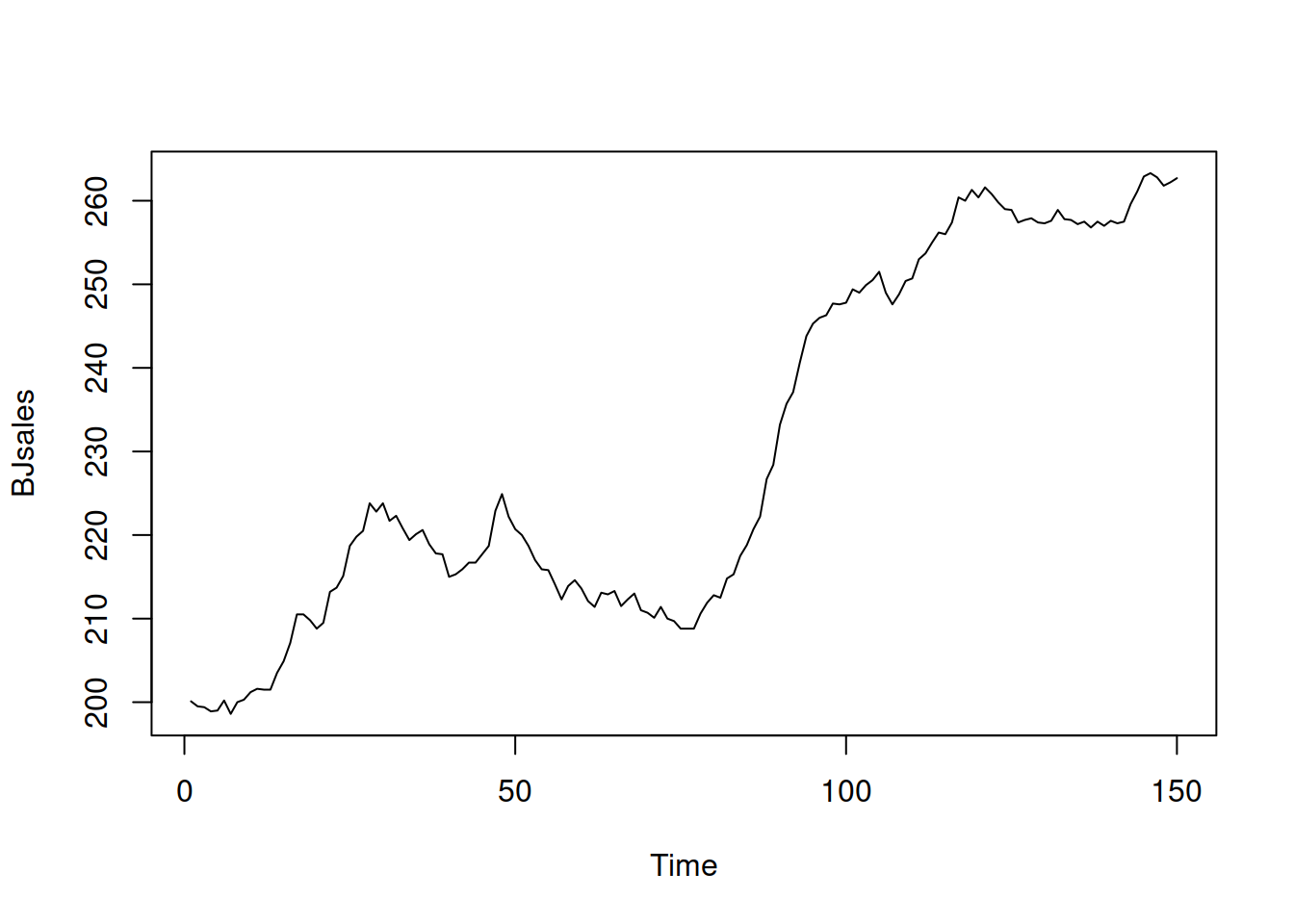Figure 5.1: Box-Jenkins sales data.

The series in Figure 5.1 seem to exhibit trend, so we will apply ETS(A,A,N) model:

adamModel <- adam(BJsales, "AAN")
adamModel
## Time elapsed: 0.03 seconds
## Model estimated using adam() function: ETS(AAN)
## Distribution assumed in the model: Normal
## Loss function type: likelihood; Loss function value: 258.8098
## Persistence vector g:
##  alpha   beta
## 1.0000 0.2438
##
## Sample size: 150
## Number of estimated parameters: 5
## Number of degrees of freedom: 145
## Information criteria:
##      AIC     AICc      BIC     BICc
## 527.6196 528.0362 542.6728 543.7166

The output of the model summarises, which specific model was estimated, assuming what distribution, how it was estimated and what the values of smoothing parameters are. It also reports the sample size, degrees of freedom and also produces (see Section 13.4 of Svetunkov, 2021c). We can compare this model with the ETS(A,N,N) in order to see, which of them performs better in terms of information criteria (e.g. in terms of AICc):

adam(BJsales, "ANN")
## Time elapsed: 0.01 seconds
## Model estimated using adam() function: ETS(ANN)
## Distribution assumed in the model: Normal
## Loss function type: likelihood; Loss function value: 273.2898
## Persistence vector g:
## alpha
##     1
##
## Sample size: 150
## Number of estimated parameters: 3
## Number of degrees of freedom: 147
## Information criteria:
##      AIC     AICc      BIC     BICc
## 552.5795 552.7439 561.6114 562.0233

In this situation the AICc for ETS(A,N,N) is higher than for ETS(A,A,N), so we should use the latter for forecasting purposes. We can produce point forecasts and prediction interval (in this example we will construct 90% and 95% ones) and plot them (Figure 5.2):

plot(forecast(adamModel, h=10,
interval="prediction", level=c(0.9,0.95)))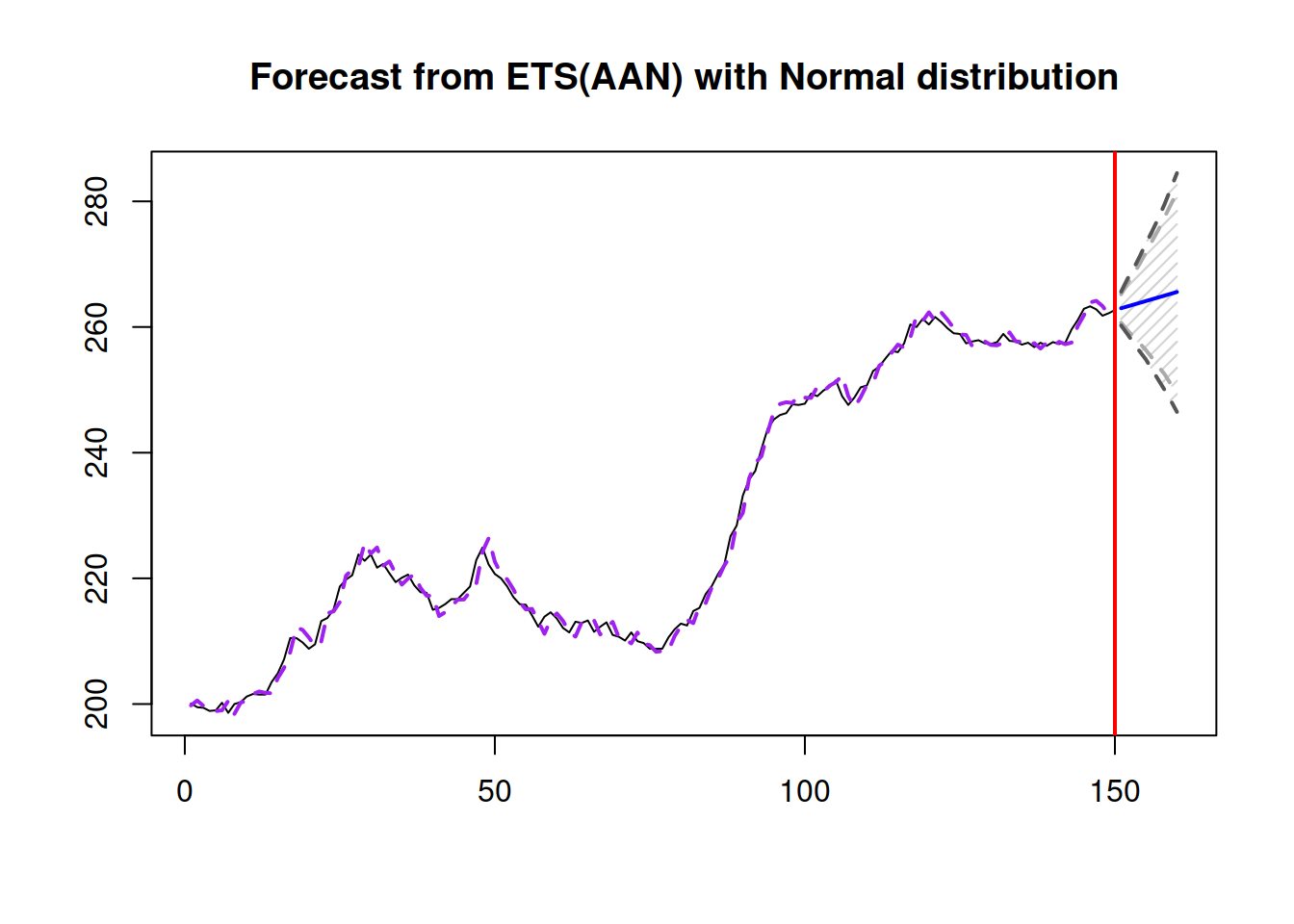Figure 5.2: Forecast for Box-Jenkins sales data from ETS(A,A,N) model.

Notice that the smoothing parameters of ETS(A,A,N) are very high, with $$\alpha=1$$. This might mean that the maximum of the likelihood is achieved in the admissible bounds. We can try it out and see what happens:

adamModel <- adam(BJsales, "AAN", bounds="admissible")
adamModel
## Time elapsed: 0.06 seconds
## Model estimated using adam() function: ETS(AAN)
## Distribution assumed in the model: Normal
## Loss function type: likelihood; Loss function value: 258.5358
## Persistence vector g:
##  alpha   beta
## 1.0541 0.2185
##
## Sample size: 150
## Number of estimated parameters: 5
## Number of degrees of freedom: 145
## Information criteria:
##      AIC     AICc      BIC     BICc
## 527.0716 527.4883 542.1248 543.1687

Both smoothing parameters are now higher, which implies that the uncertainty about the future values of states is higher as well, which is then reflected in the slightly wider prediction interval (Figure 5.3):

plot(forecast(adamModel, h=10,
interval="prediction", level=c(0.9,0.95)))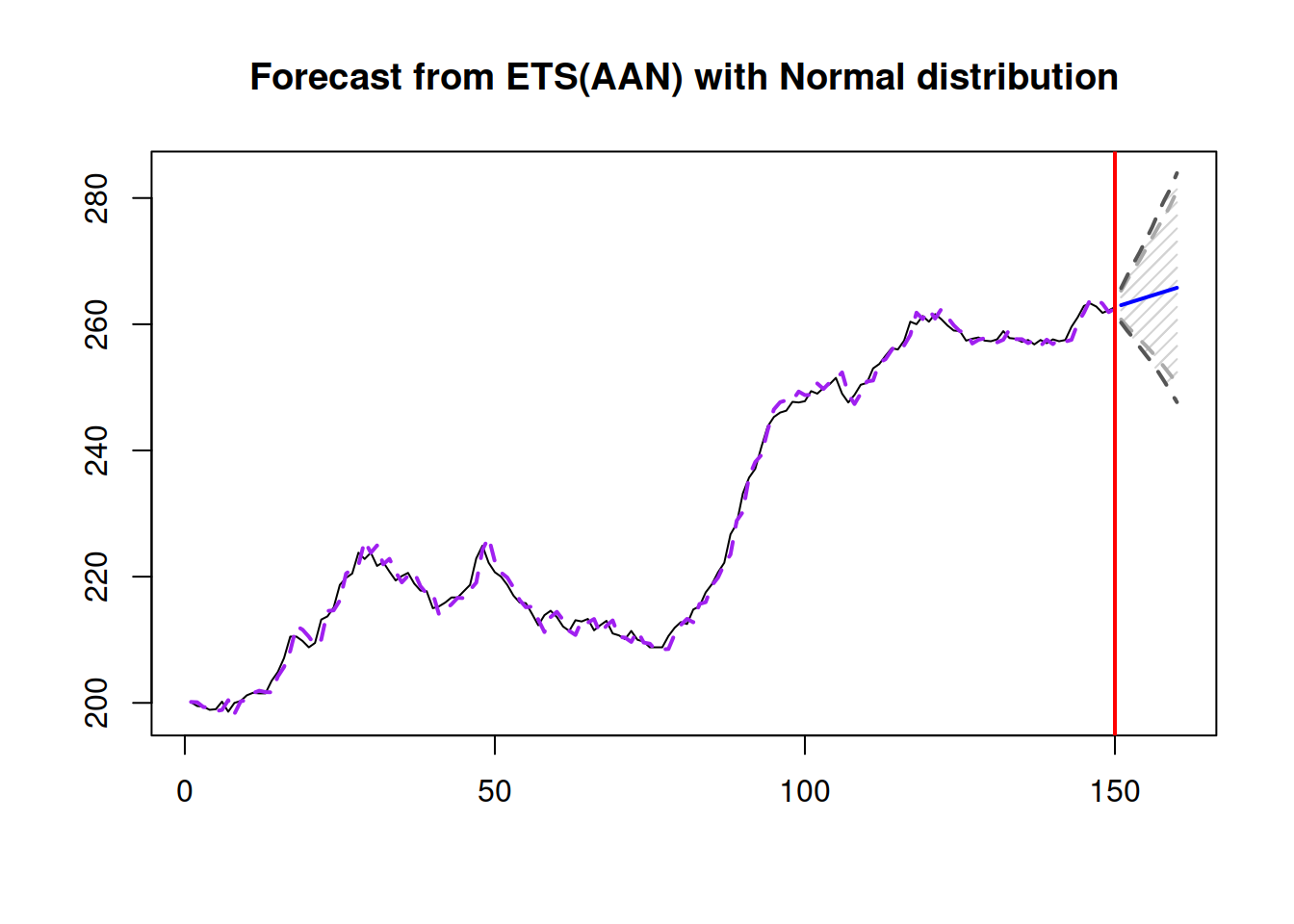Figure 5.3: Forecast for Box-Jenkins sales data from ETS(A,A,N) model with admissible bounds.

Although the values of smoothing parameters are larger than one, the model is still stable. In order to see that, we can calculate the discount matrix $$\mathbf{D}$$ using the objects returned by the function:

discountMatrix <- adamModel$transition - adamModel$persistence %*%
adamModel$measurement[nobs(adamModel),,drop=FALSE] eigen(discountMatrix)$values
##   0.79538429 -0.06800887

Notice that the absolute values of both eigenvalues in the matrix are less than one, which means that the newer observations have higher weights than the older ones and that the absolute values of weights decrease over time, making the model stable.

If we want to test ADAM ETS with another distribution, it can be done using the respective parameter (here we use Generalised Normal, estimating the shape together with the other parameters):

adamModel <- adam(BJsales, "AAN", distribution="dgnorm")
print(adamModel, digits=3)
## Time elapsed: 0.05 seconds
## Model estimated using adam() function: ETS(AAN)
## Distribution assumed in the model: Generalised Normal with shape=1.741
## Loss function type: likelihood; Loss function value: 258.456
## Persistence vector g:
## alpha  beta
## 1.000 0.217
##
## Sample size: 150
## Number of estimated parameters: 6
## Number of degrees of freedom: 144
## Information criteria:
##     AIC    AICc     BIC    BICc
## 528.913 529.500 546.977 548.448

Similar to the previous cases, we can plot the forecasts from the model:

plot(forecast(adamModel, h=10, interval="prediction"))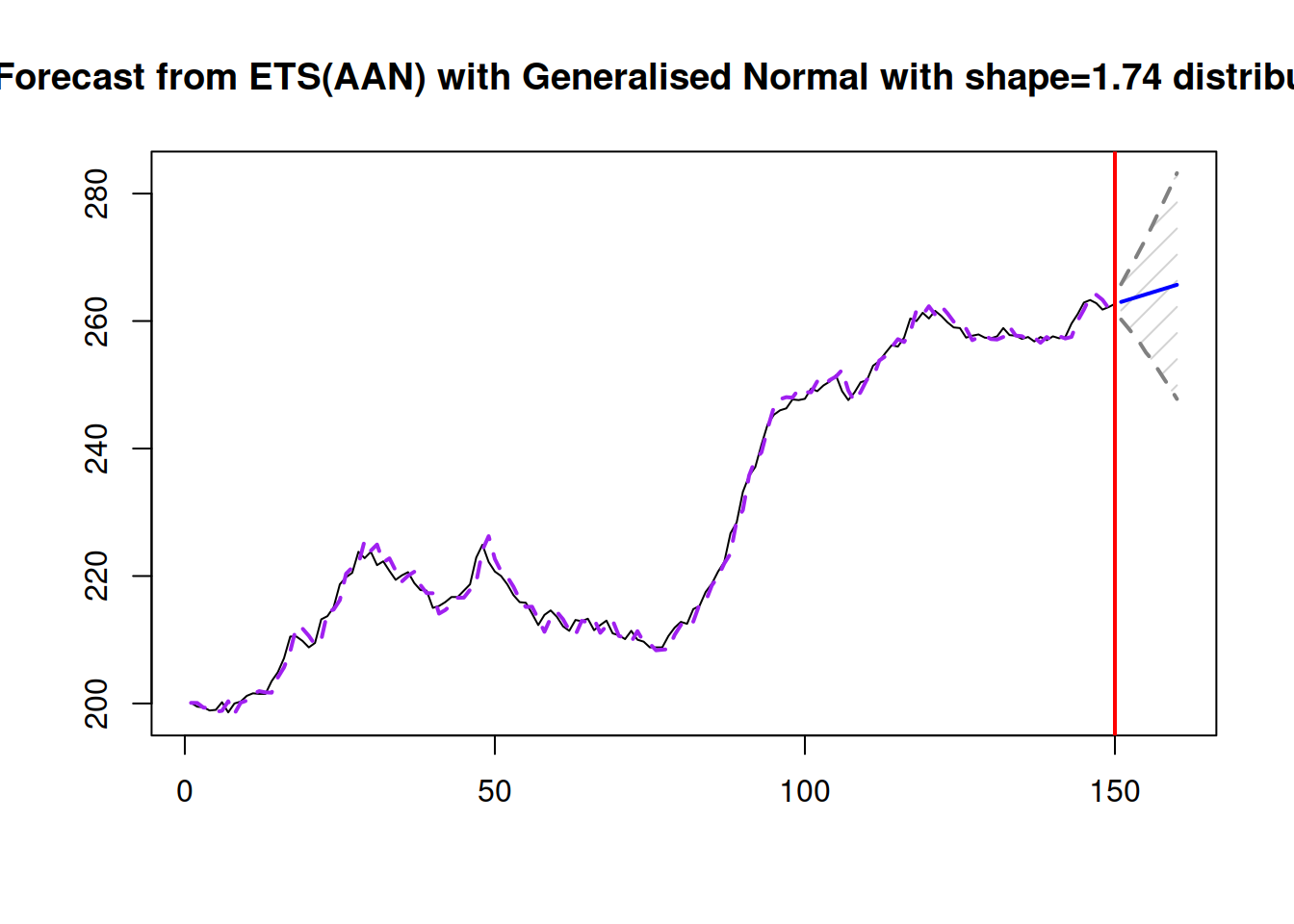Figure 5.4: Forecast for Box-Jenkins sales data from ETS(A,A,N) model with Generalised Normal distribution.

The prediction interval in this case is slightly wider than in the previous one, because $$\mathcal{GN}$$ distribution with $$\beta=$$ 1.74 has fatter tails than the normal distribution (Figure 5.4).

### 5.6.2 Seasonal data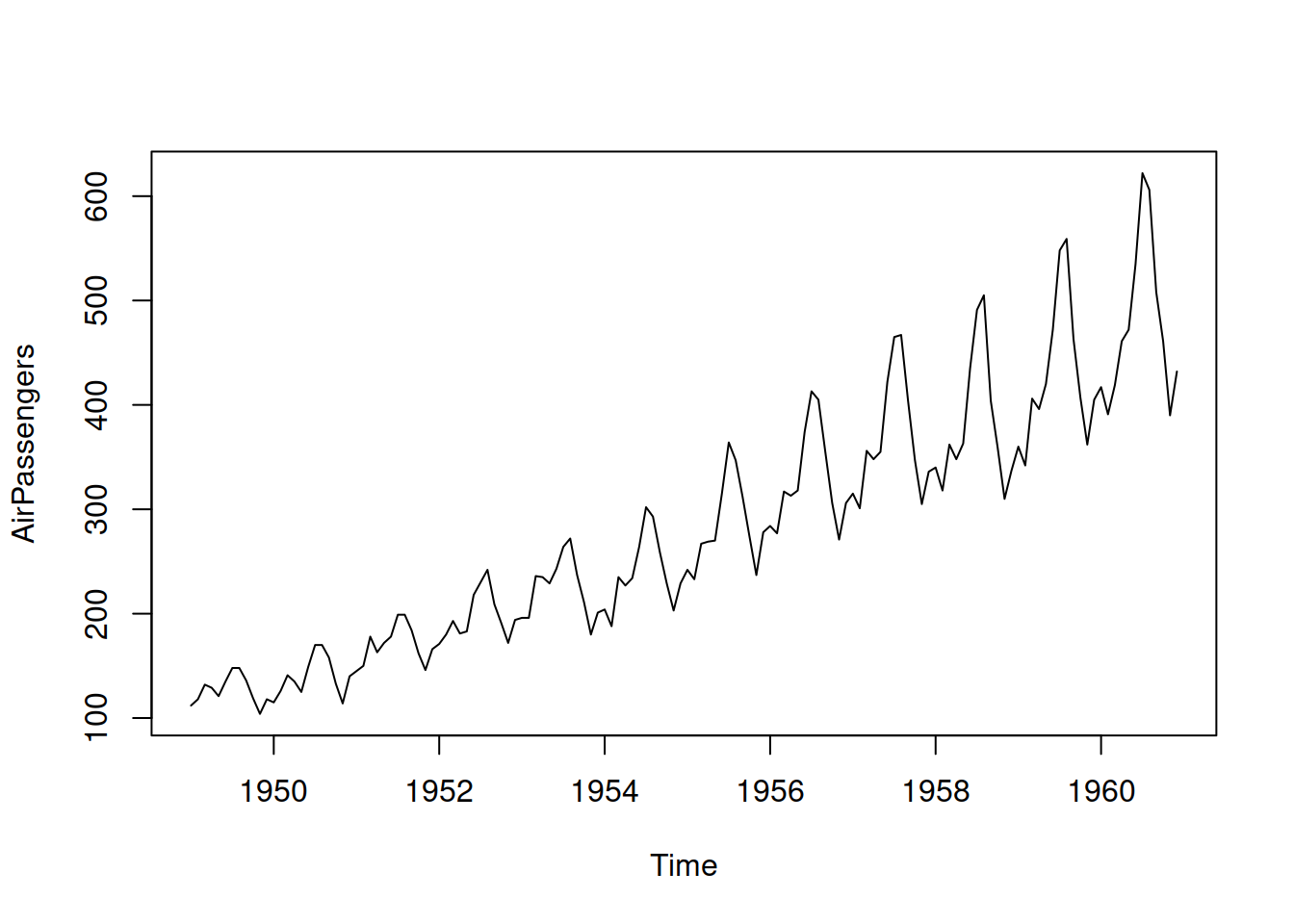Figure 5.5: Air passengers data from Box-Jenkins textbook.

Now we will check what happens in the case of seasonal data. We use AirPassengers data, plotted in Figure 5.5, which actually has multiplicative seasonality. But for purposes of demonstration, we will see what happens, when we use the wrong model with additive seasonality. We will withhold 12 observations to look closer at the performance of the ETS(A,A,A) model in this case:

adamModel <- adam(AirPassengers, "AAA", lags=12,
h=12, holdout=TRUE)

Remark. The lags parameter in this specific case is not necessary, because the function will get the frequency from the ts object automatically. If we were to provide a vector of values instead of the ts object, we would need to specify the correct lag. Note that 1 (lag for level and trend) is not needed - the function will always use it anyway.

Remark. In some cases, the optimiser might converge to the local minimum, so if you find the results unsatisfactory, it might make sense to reestimate the model tuning the parameters of the optimiser (see Section 11.4 for details). Here is an example (we increase the number of iterations in the optimisation and set new starting values for the smoothing parameters):

adamModel$B[1:3] <- c(0.2,0.1,0.3) adamModel <- adam(AirPassengers, "AAA", lags=12, h=12, holdout=TRUE, B=adamModel$B, maxeval=1000)
adamModel
## Time elapsed: 0.16 seconds
## Model estimated using adam() function: ETS(AAA)
## Distribution assumed in the model: Normal
## Loss function type: likelihood; Loss function value: 513.0026
## Persistence vector g:
##  alpha   beta  gamma
## 0.1928 0.0000 0.8072
##
## Sample size: 132
## Number of estimated parameters: 17
## Number of degrees of freedom: 115
## Information criteria:
##      AIC     AICc      BIC     BICc
## 1060.005 1065.374 1109.013 1122.119
##
## Forecast errors:
## ME: 6.216; MAE: 14.162; RMSE: 17.75
## sCE: 28.418%; Asymmetry: 51.9%; sMAE: 5.395%; sMSE: 0.457%
## MASE: 0.588; RMSSE: 0.567; rMAE: 0.186; rRMSE: 0.172

Notice that because we fit the additive seasonal model to the data with multiplicative seasonality, the smoothing parameter $$\gamma$$ has become large - the seasonal component needs to be updated in order to keep up with the changing seasonal profile. In addition, because we use holdout parameter, the function now also reports the error measures for the point forecasts on that part of data. This can be useful, when you want to compare the performance of several models on a time series. Here how the forecast from ETS(A,A,A) looks on this data: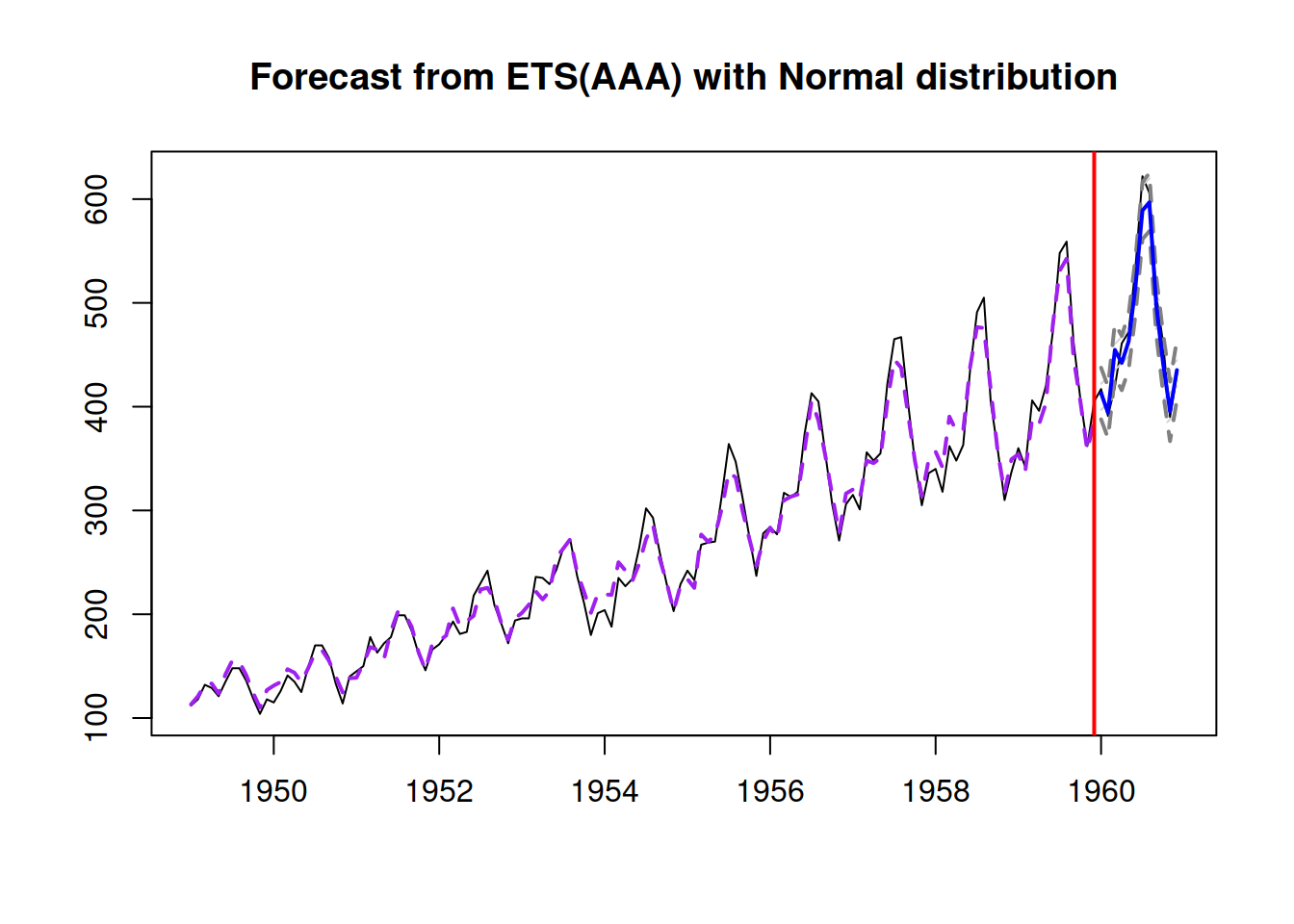Figure 5.6: Forecast for air passengers data using ETS(A,A,A) model.

Figure 5.6 demonstrates that while the fit to the data is far from perfect, due to a pure coincidence the point forecast from this model is decent.

In order to see how the ADAM ETS decomposes the data into components, we can plot it via the plot() method with which parameter: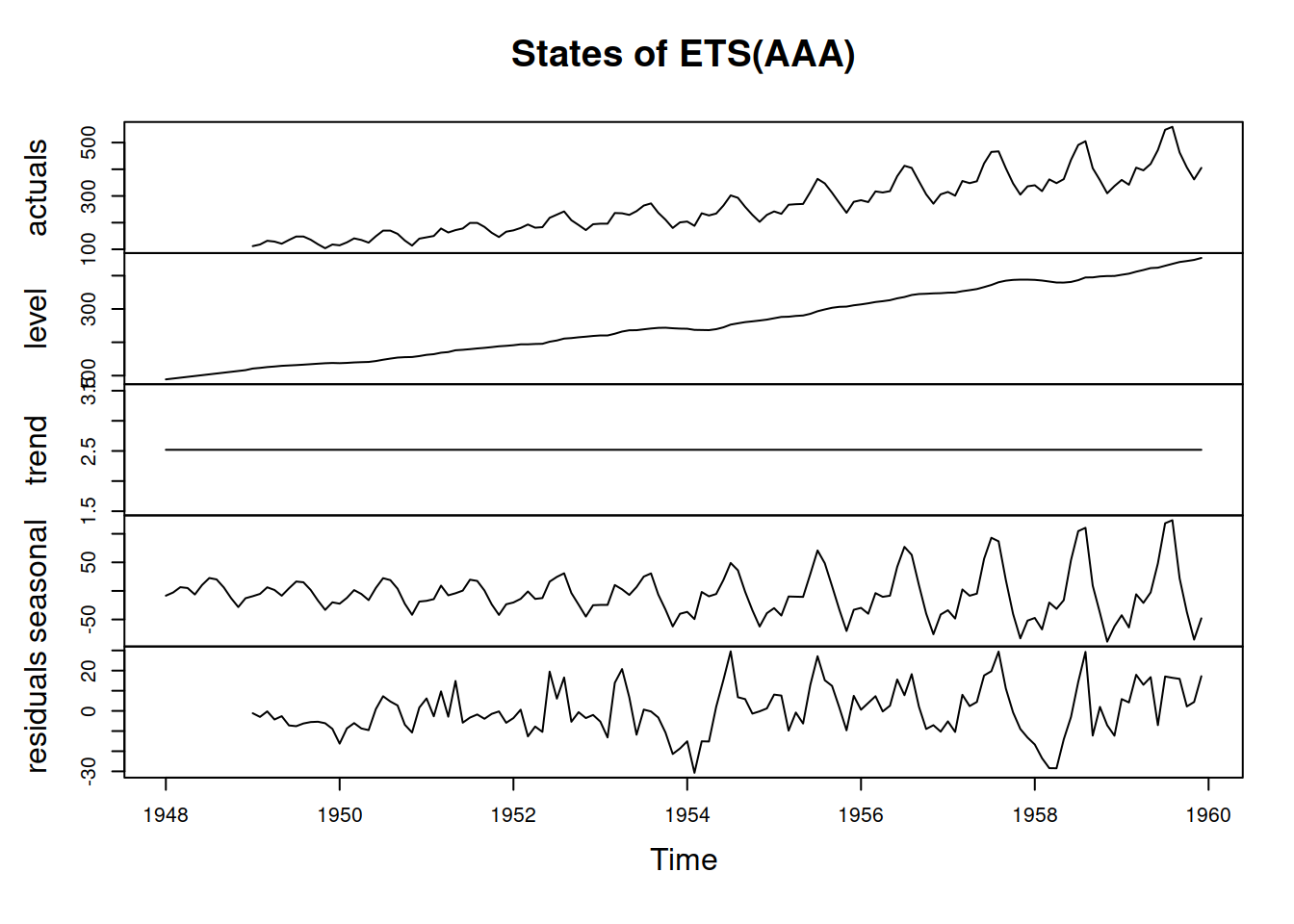Figure 5.7: Decomposition of air passengers data using ETS(A,A,A) model.

We can see on the graph in Figure 5.7 that the residuals still contain some seasonality in them, so there is a room for improvement. Most probably, this happened because the data exhibits multiplicative seasonality rather than the additive one. For now, we do not aim to fix this issue.

### References

• Svetunkov, I., 2021c. Statistics for business analytics. https://openforecast.org/sba/ (version: 01.10.2021)